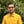Trusted answers to developer questions
Trusted Answers to Developer Questions

Related Tags

swift
acos
math
communitycreator

# What is the acos() function in Swift?Harris Amjad

Grokking Modern System Design Interview for Engineers & Managers

Ace your System Design Interview and take your career to the next level. Learn to handle the design of applications like Netflix, Quora, Facebook, Uber, and many more in a 45-min interview. Learn the RESHADED framework for architecting web-scale applications by determining requirements, constraints, and assumptions before diving into a step-by-step design process.

### Overview

In Swift, the acos() function, also called the arc cosine function, returns the inverse cosine of a number.

The image below shows the mathematical representation of the acos() function:

Mathematical representation of the inverse cosine function

Note: We need to import Foundation in our code to use the acos() function. We can import it using import Foundation.

To convert radians to degrees, use the following formula:

degrees = radians * ( 180.0 / pi )

### Syntax

acos(number)
//number can be real, float, or double.


### Parameters

This function requires a number as a parameter. The parameter must be a value between -1 and 1.

If the value is outside -1 <= parameter <= 1, acos() returns NaN.

### Return value

This function returns the inverse cosine of a number that is sent as a parameter. The return value lies in the interval [0, PI] radians.

### Example

import Swiftimport Foundation//positive number in radiansprint("The value of acos(0.5) :", acos(0.5), "Radians");// negative number in radiansprint("The value of acos(-0.5) :", acos(-0.5), "Radians");//applying acos() and then converting the result in radians to degrees// radians = 0.5// PI = 3.14159265print("The value of acos(0.5): ",acos(0.5) * (180.0 / Double.pi)," Degrees");//error outputprint("The value of acos(1.5): ",acos(1.5));print("The value of acos(-1.5): ",acos(-1.5));
Implementation of acos()

### Explanation

• Line 2: We add the Foundation header required for the acos() function.
• Line 5: We use acos() to calculate the arc cosine of the positive number.
• Line 8: We use acos() to calculate the arc cosine of the negative number.
• Line 13: We use acos() to calculate the arc cosine of a positive number using acos() and convert the result to degrees.
• Line 15–17: We use acos() to calculate the arc cosine of exceptional numbers.

RELATED TAGS

swift
acos
math
communitycreator

CONTRIBUTORHarris Amjad

Grokking Modern System Design Interview for Engineers & Managers

Ace your System Design Interview and take your career to the next level. Learn to handle the design of applications like Netflix, Quora, Facebook, Uber, and many more in a 45-min interview. Learn the RESHADED framework for architecting web-scale applications by determining requirements, constraints, and assumptions before diving into a step-by-step design process.

Keep Exploring

Learn in-demand tech skills in half the time